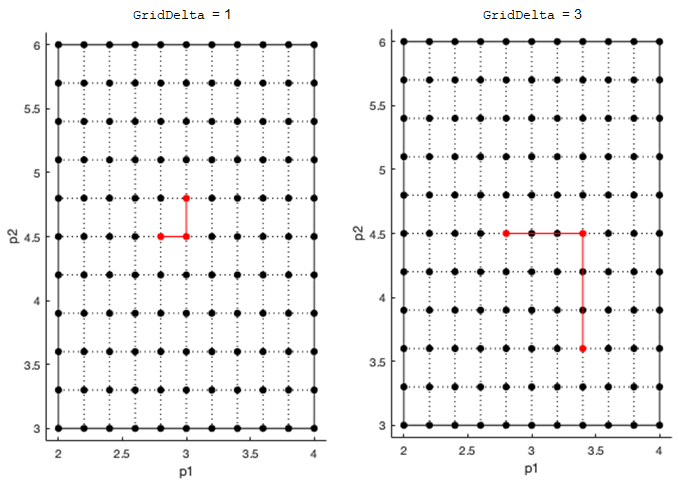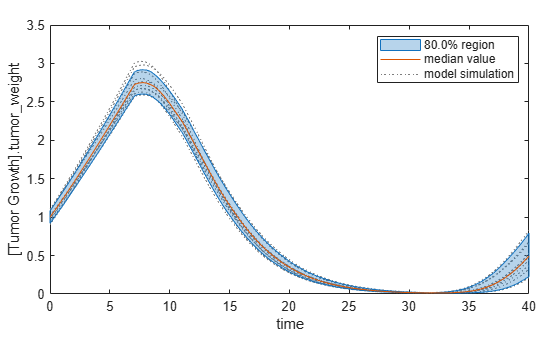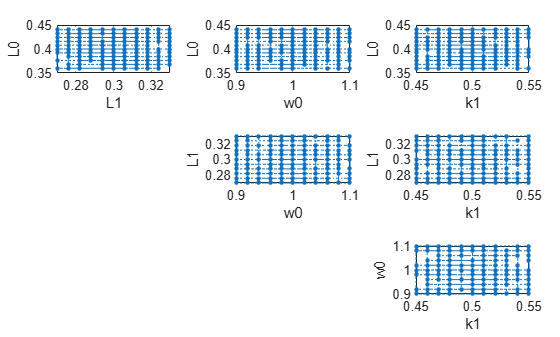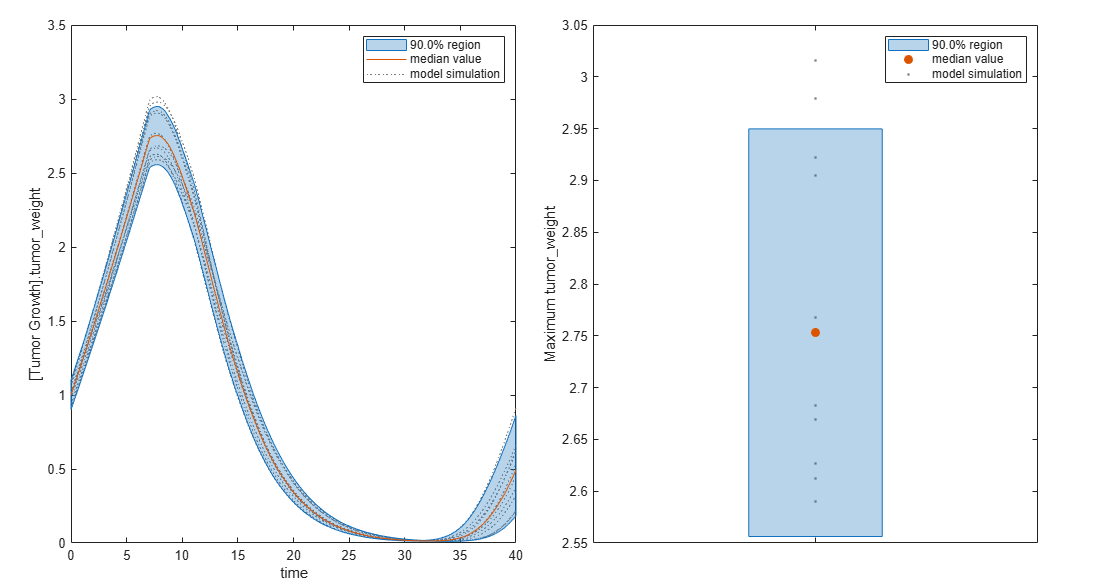SimBiology.gsa.ElementaryEffects

Object containing results from calculation of elementary effects for global sensitivity analysis (GSA)

Description

The SimBiology.gsa.ElementaryEffects object contains GSA results returned by sbioelementaryeffects. The object contains the computed elementary effects with respect to parameter inputs.

Creation

Create a SimBiology.gsa.ElementaryEffects object using sbioelementaryeffects.

Properties

expand all

Flag to use the absolute values of elementary effects, specified as true or false. By default, the function uses the absolute values of elementary effects. Using nonabsolute values can average out when calculating the mean. For details, see Elementary Effects for Global Sensitivity Analysis.

Data Types: logical

Time points at which elementary effects are computed, specified as a column numeric vector. The property is [] if all observables are scalars.

Data Types: double

GSA results with elementary effects, specified as a structure array. The size of the array is params-by-observables, where params is the number of input parameters (sensitivity inputs) and observables is the number of observables (sensitivity outputs).

Each structure contains the following fields.

• Parameter — Name of an input parameter, specified as a character vector

• Observable — Name of an observable, specified as a character vector

• Mean — Mean of absolute values of elementary effects, specified as a scalar or numeric vector

• StandardDeviation — Standard deviation of absolute elementary effects, specified as a scalar or numeric vector

If all observables are scalar, then the Mean and StandardDeviation fields are specified as scalars. If any observables are vectors, Mean and StandardDeviation are numeric vectors of length Time. If some observables are scalars and some are vectors, scalar observables are scalar-expanded, where each time point has the same value.

Data Types: struct

Discretization level of the parameter domain, specified as a positive even integer. This parameter defines a grid of equidistant points in the parameter domain, where each dimension is discretized using Gridlevel+1 points. The following figure shows an example of a grid for parameters p1 and p2 within given parameter bounds.For details, see Elementary Effects for Global Sensitivity Analysis.

Data Types: double

Step size for computing elementary effects, specified as a positive integer between 1 and GridLevel. The step size is measured in terms of grid points between neighboring points. The following figure shows examples of different grid delta values.For details, see Elementary Effects for Global Sensitivity Analysis.

Data Types: double

Method to select sample points to compute elementary effects, specified as "chain" or "radial". The "chain" point selection uses the Morris method . The "radial" point selection uses the Sohier method . For details, see Elementary Effects for Global Sensitivity Analysis.

Data Types: double

Sampled parameter values, specified as a table. The table contains (1+numel(params))*NumberSamples rows and numel(params) columns.

sbioelementaryeffects uses blocks of k+1 rows, where k is the number of input params, to compute an elementary effect for each input parameter. The total number of these blocks is equal to the total number of samples. You can get the block indices by running kron((1:NumberSamples)',ones(numel(params)+1,1)). For details, see Elementary Effects for Global Sensitivity Analysis.

Data Types: table

Names of model responses or observables, specified as a cell array of character vectors.

Data Types: char

Simulation information, such as simulation data and parameter samples, used for computing elementary effects, specified as a structure. The structure contains the following fields.

• SimFunctionSimFunction object used for simulating model responses or observables.

• SimDataSimData array of size [NumberSamples,1], where NumberSamples is the number of samples. The array contains simulation results from ParameterSamples.

• OutputTimes — Numeric column vector containing the common time vector of all SimData objects.

• Bounds — Numeric matrix of size [params,2]. params is the number of input parameters. The first column contains the lower bounds and the second column contains the upper bounds for sensitivity inputs.

• DoseTables — Cell array of dose tables used for the SimFunction evaluation. DoseTables is the output of getTable(doseInput), where doseInput is the value specified for the 'Doses' name-value pair argument in the call to sbiosobol, sbiompgsa, or sbioelementaryeffects. If no doses are applied, this field is set to [].

• ValidSample — Logical vector of size [NumberSamples,1] indicating whether a simulation for a particular sample failed. Resampling of the simulation data (SimData) can result in NaN values if the data is extrapolated. Such SimData are indicated as invalid.

• InterpolationMethod — Name of the interpolation method for SimData.

• SamplingMethod — Name of the sampling method used to draw ParameterSamples.

• RandomState — Structure containing the state of rng before drawing ParameterSamples.

Data Types: struct

Object Functions

 resample Resample Sobol indices or elementary effects to new time vector addobservable Compute Sobol indices or elementary effects for new observable expression removeobservable Remove Sobol indices or elementary effects of observables addsamples Add additional samples to increase accuracy of Sobol indices or elementary effects analysis plotData Plot quantile summary of model simulations from global sensitivity analysis (requires Statistics and Machine Learning Toolbox) plot Plot means and standard deviations of elementary effects bar Plot magnitudes of means and standard deviations of elementary effects plotGrid Plot parameter grid and points used to compute elementary effects

Examples

collapse all

Get a variant with estimated parameters and the dose to apply to the model.

v = getvariant(m1);
d = getdose(m1,'interval_dose');

Get the active configset and set the tumor weight as the response.

cs = getconfigset(m1);
cs.RuntimeOptions.StatesToLog = 'tumor_weight';

Simulate the model and plot the tumor growth profile.

sbioplot(sbiosimulate(m1,cs,v,d));Perform global sensitivity analysis (GSA) on the model to find the model parameters that the tumor growth is sensitive to.

First, define model parameters of interest, which are involved in the pharmacodynamics of the tumor growth. Define the model response as the tumor weight.

modelParamNames = {'L0','L1','w0','k1'};
outputName = 'tumor_weight';

Then perform GSA by computing the elementary effects using sbioelementaryeffects. Use 100 as the number of samples and set ShowWaitBar to true to show the simulation progress.

rng('default');
eeResults = sbioelementaryeffects(m1,modelParamNames,outputName,Variants=v,Doses=d,NumberSamples=100,ShowWaitbar=true);

Show the median model response, the simulation results, and a shaded region covering 90% of the simulation results.

plotData(eeResults,ShowMedian=true,ShowMean=false);You can adjust the quantile region to a different percentage by specifying Alphas for the lower and upper quantiles of all model responses. For instance, an alpha value of 0.1 plots a shaded region between the 100*alpha and 100*(1-alpha) quantiles of all simulated model responses.

plotData(eeResults,Alphas=0.1,ShowMedian=true,ShowMean=false);Plot the time course of the means and standard deviations of the elementary effects.

h = plot(eeResults);
% Resize the figure.
h.Position(:) = [100 100 1280 800];The mean of effects explains whether variations in input parameter values have any effect on the tumor weight response. The standard deviation of effects explains whether the sensitivity change is dependent on the location in the parameter domain.

From the mean of effects plots, parameters L1 and w0 seem to be the most sensitive parameters to the tumor weight before the dose is applied at t = 7. But, after the dose is applied, k1 and L0 become more sensitive parameters and contribute most to the after-dosing stage of the tumor weight. The plots of standard deviation of effects show more deviations for the larger parameter values in the later stage (t > 35) than for the before-dose stage of the tumor growth.

You can also display the magnitudes of the sensitivities in a bar plot. Each color shading represents a histogram representing values at different times. Darker colors mean that those values occur more often over the whole time course.

bar(eeResults);You can also plot the parameter grids and samples used to compute the elementary effects.

plotGrid(eeResults)You can specify more samples to increase the accuracy of the elementary effects, but the simulation can take longer to finish. Use addsamples to add more samples.

The SimulationInfo property of the result object contains various information for computing the elementary effects. For instance, the model simulation data (SimData) for each simulation using a set of parameter samples is stored in the SimData field of the property. This field is an array of SimData objects.

eeResults2.SimulationInfo.SimData

SimBiology SimData Array : 1500-by-1

Index:    Name:         ModelName:         DataCount:
1           -           Tumor Growth Model 1
2           -           Tumor Growth Model 1
3           -           Tumor Growth Model 1
...
1500        -           Tumor Growth Model 1

You can find out if any model simulation failed during the computation by checking the ValidSample field of SimulationInfo. In this example, the field shows no failed simulation runs.

all(eeResults2.SimulationInfo.ValidSample)
ans = logical
1

You can add custom expressions as observables and compute the elementary effects of the added observables. For example, you can compute the effects for the maximum tumor weight by defining a custom expression as follows.

% Suppress an information warning that is issued.
warnSettings = warning('off', 'SimBiology:sbservices:SB_DIMANALYSISNOTDONE_MATLABFCN_UCON');

Plot the computed simulation results showing the 90% quantile region.

h2 = plotData(eeObs);
h2.Position(:) = [100 100 1500 800];You can also remove the observable by specifying its name.

eeNoObs = removeobservable(eeObs,'Maximum tumor_weight');

Restore the warning settings.

warning(warnSettings);

 Morris, Max D. “Factorial Sampling Plans for Preliminary Computational Experiments.” Technometrics 33, no. 2 (May 1991): 161–74.

 Sohier, Henri, Jean-Loup Farges, and Helene Piet-Lahanier. “Improvement of the Representativity of the Morris Method for Air-Launch-to-Orbit Separation.” IFAC Proceedings Volumes 47, no. 3 (2014): 7954–59.

SimBiology DocumentationTry MATLAB, Simulink, and Other Products

Get trial now